• 用于matlab中图片的ROI区域提取，若对彩色图片的处理需要改一改，只是爲了方便，不喜勿噴。。。。。。。
• 2] #print(src.shape) ####################################################提取ROI区域 cut=src[40:950,90:1200] plt.imshow(cut) plt.show() ''' cv.imshow('cut', cut) cv.waitKey(0) ''' # 手工绘制ROI区域 ...
 代码里面说的比较明白

思路很简单，就是选取多边形顶点，制作掩膜mask，然后用掩膜与图片做按位与操作，就可以得到ROI区域。

import numpy as np
import cv2 as cv
from matplotlib import pyplot as plt

if __name__=='__main__':
original = cv.imread('./test_img/line/line7.jpg')
src=cv.imread('./test_img/line/line7.jpg',0)
(h,w)=src.shape[:2]
#print(src.shape)

####################################################提取ROI区域
cut=src[40:950,90:1200]

plt.imshow(cut)
plt.show()
'''
cv.imshow('cut', cut)
cv.waitKey(0)
'''
# 手工绘制ROI区域
mask = np.zeros((cut.shape), dtype=np.uint8)
#注意numpy的坐标系和plt的坐标系一样，别搞错了！都是y朝下，x朝右；而cv是x朝下，y朝右！！！所以下面得numpy数组坐标按照plt下即可，无需对调！
#多边形顶点坐标，x,y上下对应
x_data = np.array([0,0,770,1108,1108,770])
y_data = np.array([0,900,900,700,190,10])
'''
矩阵.T 就是对一个矩阵转置
'''
# 按垂直方向（行顺序）堆叠数组构成一个新的数组,堆叠的数组需要具有相同的维度
pts = np.vstack((x_data, y_data)).astype(np.int32).T
#其实上面就是为了变到下面这种形式
#pts=np.array([[0,0],[0,900],[700,900],[1108,700],[1108,190],[770,10]])
#print(pts)

'''
函数cvFillPoly用于一个单独被多边形轮廓所限定的区域内进行填充。函数可以填充复杂的区域,例如，有漏洞的区域和有交叉点的区域等等。
color :多边形的颜色。
line_type :组成多边形的线条的类型。
shift :顶点坐标的小数点位数。
注意：pts外面要加[]变成三维数组
'''
#生成的掩膜mask是二值图像
cv.fillPoly(img=mask,pts=[pts],color=(255,0,0),lineType=8,shift=0)
cv.imshow("mask", mask)
cv.waitKey(0)
#print(mask)
#print(mask.shape)
#print(cut.shape)
# 根据mask，提取ROI区域
'''
按位与：因为掩膜图片中ROI区域的白色部分，即255（二进制是11111111，故与此部分相与仍是原数，
但非ROI区域是黑色，即00000000，与全0相与那么就会变成0，即不感兴趣的部分就会变成黑色
'''
#参数意义：就是src1和src2这两个数据都要先和mask按位与，然后再按位与， 如果有mask这个参数的话
ROI= cv.bitwise_and(src1=cut,src2=mask, mask=None)
#ROI = cv.bitwise_and(src1=cut, src2=cut, mask=mask)
cv.imshow("ROI", ROI)
cv.waitKey(0)



运行效果：

原图：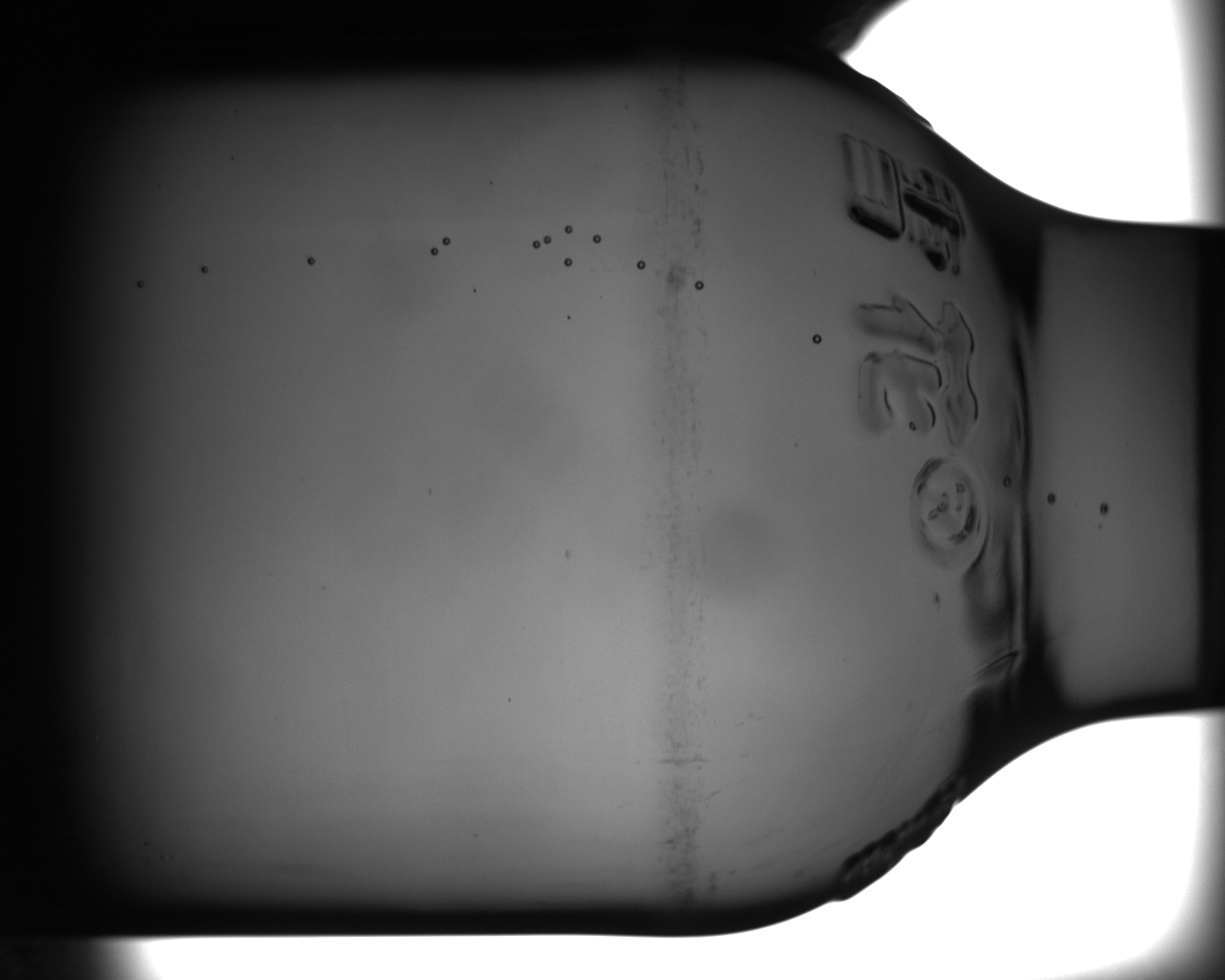掩膜：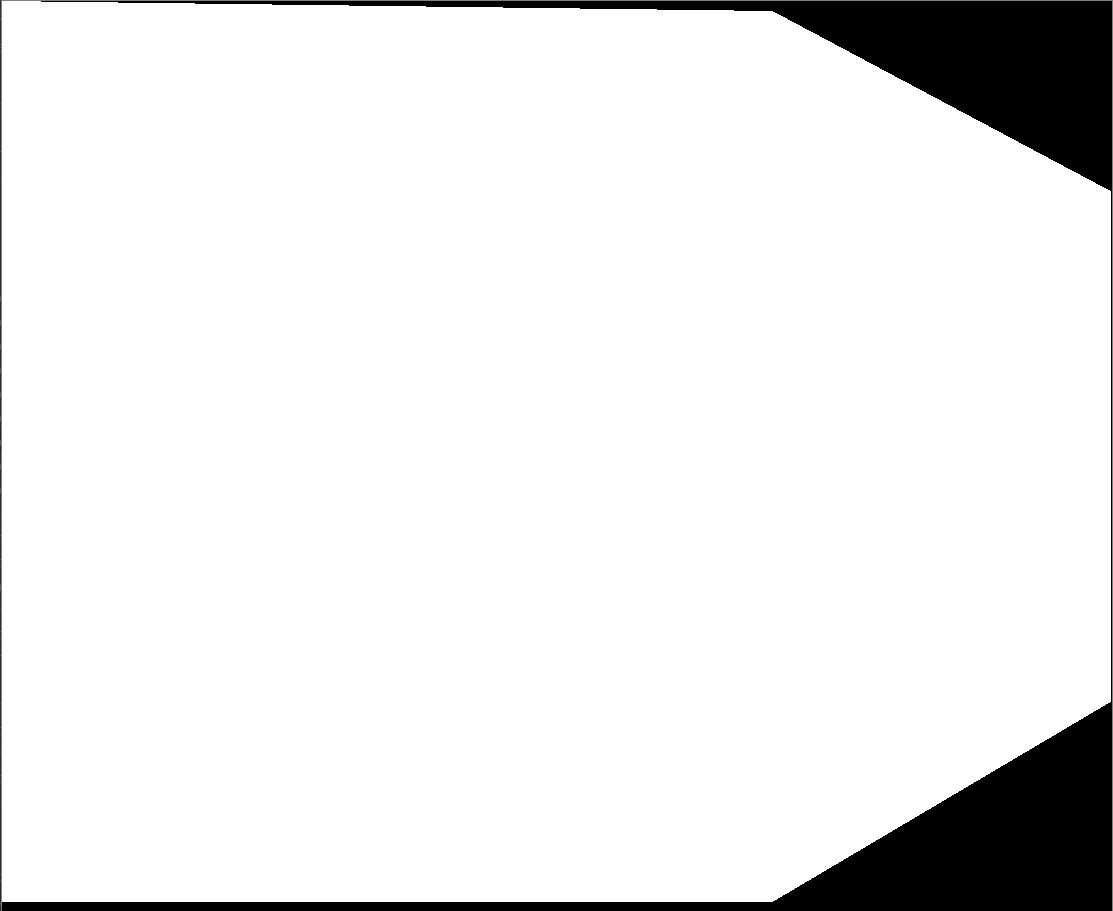ROI图片：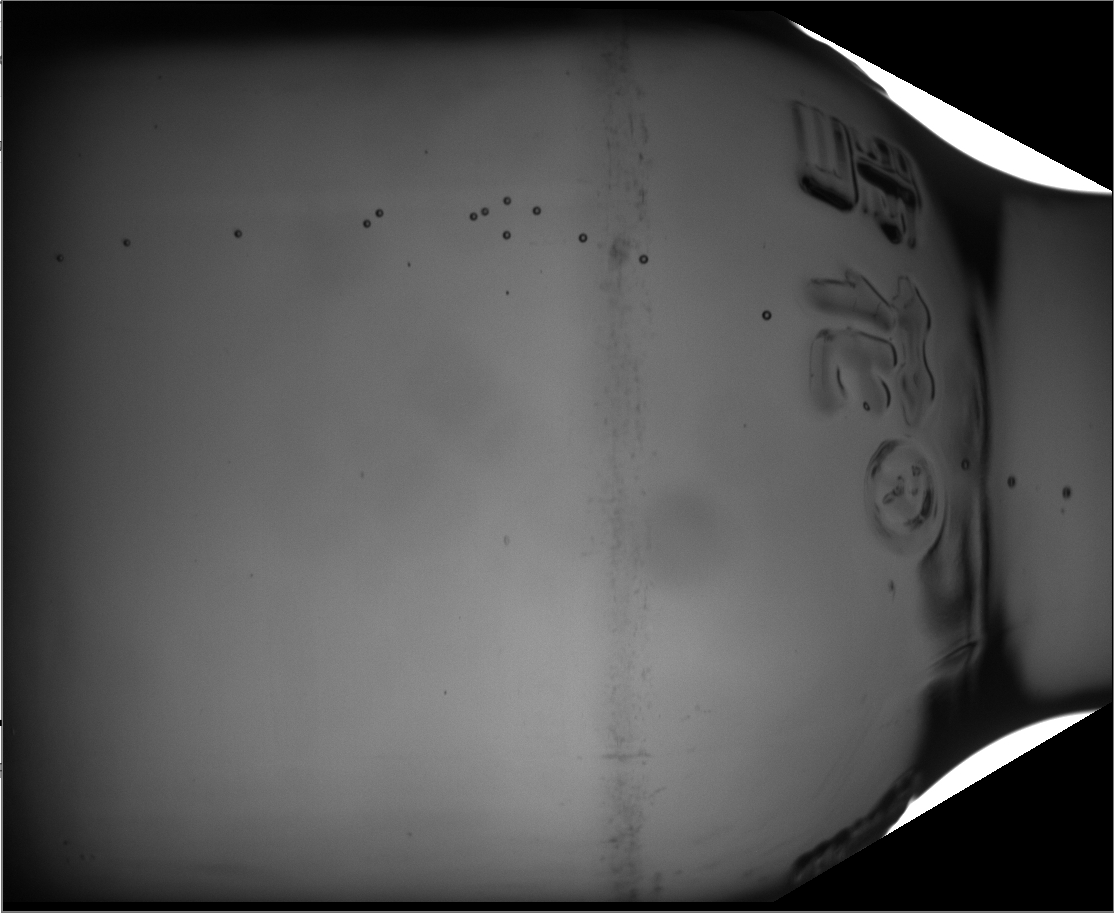若用inRange函数则不行，因为灰度值差异不大。。无法限定有效范围


展开全文• 下面是ROI区域坐标提取代码讲解，这段代码利用鼠标事件可以在照片上或者是视频中截取的某帧图像上的图片进行划分。 导入库： import cv2 import numpy as np import joblib 建立空的列表，用于存放点坐标。 pts = []...
下面是ROI区域坐标提取代码讲解，这段代码利用鼠标事件可以在照片上或者是视频中截取的某帧图像上的图片进行划分。
import cv2
import numpy as np
import joblib

pts = []  # 建立空的列表，用于存放点坐标

def draw_roi(event, x, y, flags, param):
img2 = img.copy()

if event == cv2.EVENT_LBUTTONDOWN:  # 左键点击，选择点
pts.append((x, y))
if event == cv2.EVENT_RBUTTONDOWN:  # 右键点击，取消最近一次选择的点
pts.pop()
if event == cv2.EVENT_MBUTTONDOWN:  # 中键绘制轮廓
mask = np.zeros(img.shape, np.uint8)
points = np.array(pts, np.int32)
points = points.reshape((-1, 1, 2))

# 画多边形
mask = cv2.polylines(mask, [points], True, (255, 255, 255), 2)
mask2 = cv2.fillPoly(mask.copy(), [points], (255, 255, 255))  # 用于求 ROI
mask3 = cv2.fillPoly(mask.copy(), [points], (0, 255, 0))      # 用于 显示在桌面的图像
show_image = cv2.addWeighted(src1=img, alpha=0.8, src2=mask3, beta=0.2, gamma=0)
cv2.imshow("mask", mask2)
cv2.imshow("show_img", show_image)
ROI = cv2.bitwise_and(mask2, img)
cv2.imshow("ROI", ROI)
cv2.waitKey(0)

if len(pts) > 0:
# 将pts中的最后一点画出来
cv2.circle(img2, pts[-1], 3, (0, 0, 255), -1)
if len(pts) > 1:
# 画线
for i in range(len(pts) - 1):
cv2.circle(img2, pts[i], 5, (0, 0, 255), -1)  # x ,y 为鼠标点击地方的坐标
cv2.line(img=img2, pt1=pts[i], pt2=pts[i + 1], color=(255, 0, 0), thickness=2)
cv2.imshow('image', img2)

# 创建图像与窗口并将窗口与回调函数绑定
path=r"E:\yolov4-deepsort-master\data\video\4.png" #修改路径

#为了使ROI与实际的点的坐标一致，需要将图片resize成目标大小，这里我是在视频中画ROI，所以为了匹配大小重新改了图片大小
img_org = cv2.imread(path)
print('img_org.size:',img_org.shape)
img=cv2.resize(img_org,(1920,1080))
print('img.size:',img.shape)

cv2.namedWindow('image')
cv2.setMouseCallback('image', draw_roi)
print("[INFO] 单击左键：选择点，单击右键：删除上一次选择的点，单击中键：确定ROI区域")
print("[INFO] 按‘S’确定选择区域并保存")
print("[INFO] 按 ESC 退出")

#退出与保存
while True:
key = cv2.waitKey(1) & 0xFF
if key == 27:
break
if key == ord("s"):
saved_data = {"ROI": pts}
joblib.dump(value=saved_data, filename="config.pkl")
print("[INFO] ROI坐标已保存到本地.")
break
cv2.destroyAllWindows()

#加载保存好的坐标
def Load_Model(filepath):
img = cv2.imread(path)
model = joblib.load(filepath)
print(type(model))
print(model)
return model
Load_Model('config.pkl')

以上代码实现ROI的坐标的提取。下面的代码将ROI提取出的坐标进行拟合，方便对图上目标位置与roi进行比对，方便后续操作：
def line(name,x1,y1,x2,y2):
k=(y1-y2)/(x1-x2)
b=y1-((y1-y2)/(x1-x2))*x1
print('L%s:y=%.2f*x+%.2f'%(name,k,b))

#下面将上述提取出的坐标进行拟合，
line('ab',0, 280,461, 180)
line('bc',0, 493,947, 315)
line('cd',947,315,1769, 399)
line('da2',1769, 399,0,1078)
line('停止线',1301,314,1505,333)
line('停止线2',1505,333,1913,270)
这里后面有时间可以封装一个函数，直接在ROI提取好坐标点以后，直接将所要拟合的坐标导入到拟合函数中，省去自己手动输入坐标的繁琐操作。


展开全文python
• 这次整理的内容是：几何形状绘制和ROI(region of interest)感兴趣区域提取。 几何形状绘制 在对图像进行各种处理时，有时候会需要将某个区域标记出来，就可以通过几何形状绘制来实现。几何形状有很多种，我就以最...
这次整理的内容是：几何形状绘制和ROI(region of interest)感兴趣区域提取。

几何形状绘制
在对图像进行各种处理时，有时候会需要将某个区域标记出来，就可以通过几何形状绘制来实现。几何形状有很多种，我就以最常见的矩形和线段来举例子，代码如下：

	RNG rng;				//一个随机数对象
image = Scalar(0, 0, 0);
int height = image.rows;
int width = image.cols;
for (int i = 0; i < 100000; i++)
{
int x1 = rng.uniform(0, width);			//rng.uniform(a, b)生成[a, b)之间的随机数，包含a，但是不包含b
int y1 = rng.uniform(0, height);
int x2 = rng.uniform(0, width);
int y2 = rng.uniform(0, height);

int R = rng.uniform(0, 256);
int G = rng.uniform(0, 256);
int B = rng.uniform(0, 256);

//LINE_AA：反锯齿绘制；LINE_4：4邻域绘制；LINE_8：8领域绘制；shift：相对位移
//line(image, Point(x1, y1), Point(x2, y2), Scalar(B, G, R), 2, LINE_AA, 0);

Rect rect;
rect.x = x1;
rect.y = y1;
rect.height = y2 - y1;
rect.width = x2 - x1;
rectangle(image, rect, Scalar(B, G, R),  1, LINE_8, 0);			//thickness(线宽)=-1：对图像进行填充；

char ch = waitKey(20);
imshow("image", image);
if (ch == 27)
{
break;
}
}

首先是定义了一个随机数对象（RNG），不是电子竞技啊亲！！！
用这个RNG对象来随机生成绘制几何形状的坐标和颜色。
然后定义一张全黑的画布，作为绘制的区域。
再使用rng.uniform(a, b)分别生成随机坐标和RGB值，注意该函数生成[a, b)之间的随机数，包含a，但是不包含b。
如果绘制线段的话，就使用line(image, Point(x1, y1), Point(x2, y2), Scalar(B, G, R), 2, LINE_AA, 0)，其中第一个参数就是画布；第二个参数是线段起点；第三个参数是线段的终点；第四个参数是线段的颜色，用函数Scalar()来赋值；第五个参数是线段的宽度；第六个参数是绘制方式，有以下几种方式 { LINE_AA：反锯齿绘制；LINE_4：4邻域绘制；LINE_8：8领域绘制 } ；第七个参数
shift表示相对位移，一般取0。
如果绘制矩形，就得先定义一个Rect类对象并初始化，再用rectangle(image, rect, Scalar(B, G, R), 1, LINE_8, 0)来绘制，其参数和绘制线段的函数是大同小异的。如果要对矩形进行填充，只需要将参数thickness(线宽) = -1 就可以了。
由于我这里使用了for循环绘制很多很多的矩形或线段，所以看起来有些眼花缭乱，效果如下图：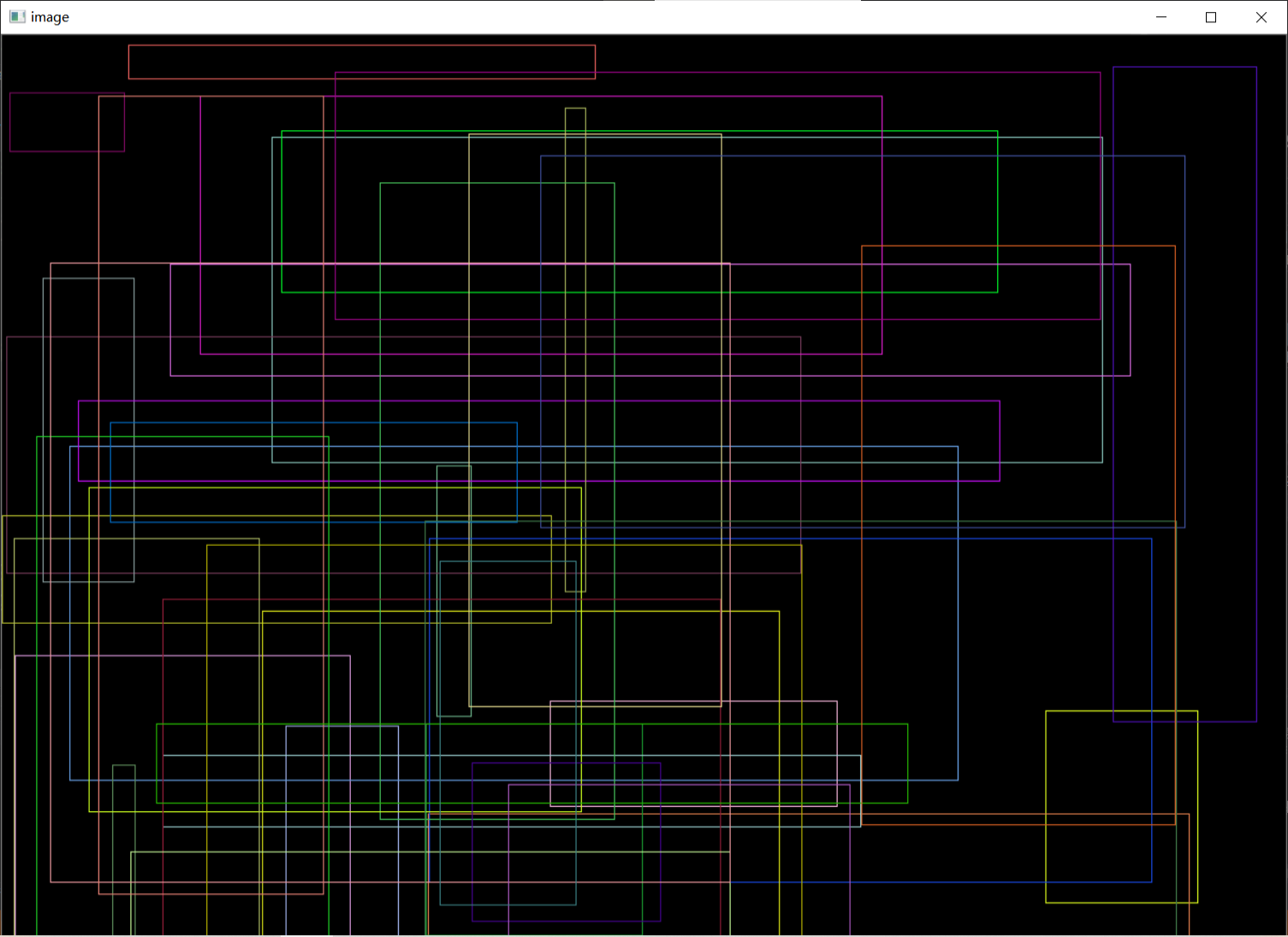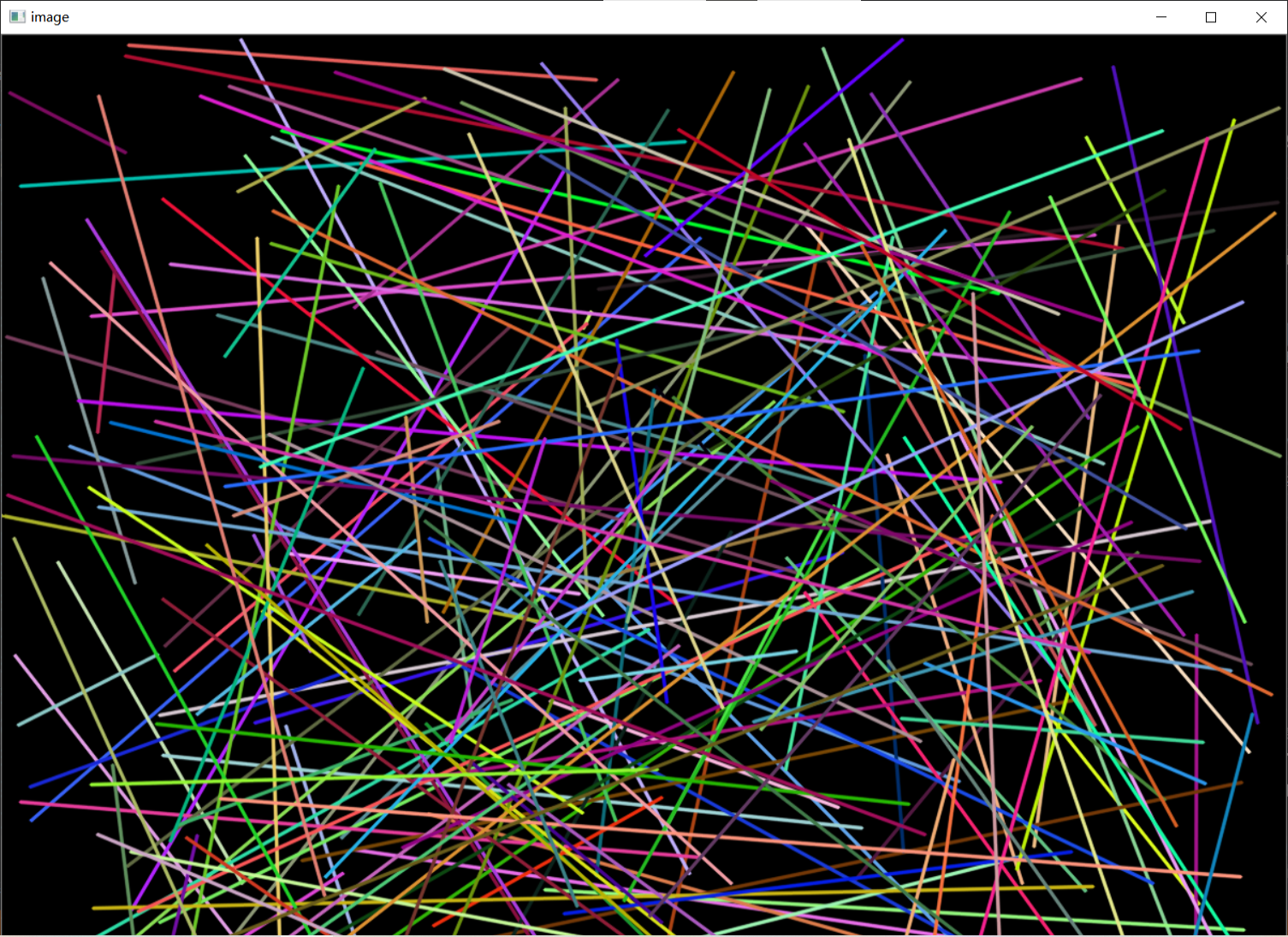2. ROI(region of interest)感兴趣区域提取
	int width = image.cols;
int height = image.rows;
int x = width / 2 - 50;
int y = height / 2 - 50;

Rect rect(x, y, x+50, y+50);
mat roi;
roi = image(rect);					//将区域提取出来，但该区域仍然指向原图
roi = image(rect).clone();			//复制成新图像， 不指向原图

roi.setto(scalar(0, 0, 0));
imshow("roi", roi);

ROI区域也就是我们所感兴趣的区域，有时候我们只是想对某个区域进行处理而不对整幅图像，这时候就需要通过ROI提取来得到我们要的处理区域。在前面我们绘制几何形状的基础上，我们就可以将绘制了的矩形区域从图像中提取出来。
当我们已经拥有了一个矩形rect时，可以通过roi = image(rect)或者roi = image(rect).clone()来将图像中的该区域提取到Mat对象roi中。要注意的是，如果使用第一个方式提取区域，那么roi表示的区域仍然是指向原图的，如果对roi进行修改，原图也会被修改；如果用第二种方式提取区域，那么roi就是一幅新的与原图无关的图像，例如将roi置为全黑的图像，原图是不会受到影响的。
同样我们可以根据自己的需求来选择使用哪种方法进行ROI区域提取。
代码中roi.setto(scalar(0, 0, 0))这一行就是将一幅图像置为单一的颜色，具体颜色由Scalar(B、G、R)来确定。
需要注意的是，当我们提取出图像的roi区域后，如果要将这个区域显示为另一张图像（例如将两张图像合并，创建输出图像dst后要把其中一部分显示一张图像，另一部分显示另一张图像），需要使用Mat().copyto()来将显示图像复制给roi区域。不能使用直接赋值的方式或者Mat().clone()方法来进行赋值，否则相当于将roi区域重新生成一张图像，不会修改输出图像dst中的内容！！！
本次整理结束，下次再继续~
PS：本人的注释比较杂，既有自己的心得体会也有网上查阅资料时摘抄下的知识内容，所以如有雷同，纯属我向前辈学习的致敬，如果有前辈觉得我的笔记内容侵犯了您的知识产权，请和我联系，我会将涉及到的博文内容删除，谢谢！


展开全文opencv c++ 计算机视觉
• ## OpenCV数字图像处理之ROI区域的提取

万次阅读 多人点赞 2019-03-04 11:07:18
OpenCV数字图像处理之ROI区域提取 利用mask(掩模)技术提取纯色背景图像ROI区域中的人和物，并将提取出来的人或物添加在其他图像上。 1、实现原理 先通过cv.cvtColor()函数，将原RGB彩色图像转换为hsv色彩空间的...
OpenCV数字图像处理之ROI区域的提取
利用mask(掩模)技术提取纯色背景图像ROI区域中的人和物，并将提取出来的人或物添加在其他图像上。
1、实现原理
先通过cv.cvtColor()函数，将原RGB彩色图像转换为hsv色彩空间的图像，然后通过cv.inRange()函数获得ROI区域的Mask，最后利用cv.bitwise()函数提取得到ROI区域。
2、使用的函数简述
(1) cv.cvtColor(img, cv.COLOR_BGR2HSV)函数
img为要进行色彩空间转换的原图
cv.COLOR_BGR2HSV即将原图RGB色彩空间转换为HSV色彩空间
(2) cv.inRange(hsv, (h_min, s_min, v_min), (h_max, s_max, v_max))函数
cv.inRange函数通过设置不同的h、s、v的min和max阈值可以获取不同色彩的一个二值的mask图，下图为各颜色的阈值表：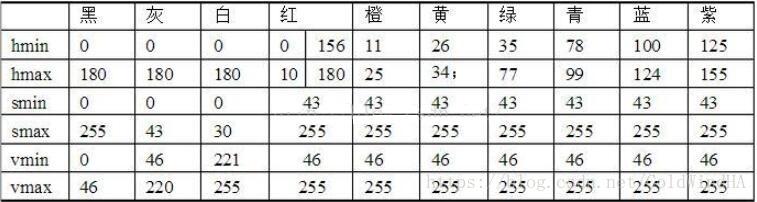(3)cv.bitwise_and(img1, img2, mask)，cv.bitwise_or(img1, img2, mask)和cv.bitwise_not(img)
第一个函数为按位与操作函数，将img1和img2在mask的区域内，R,G,B三个分量分别进行按位与操作。第二个函数为按位或操作函数，将img1和img2在mask的区域内，R,G,B三个分量分别进行按位或操作。第三个函数为按位取反操作函数，将img在R,G,B三个分量分别进行按位取反操作。
(4)cv.add(img1, img2)函数
将img1和img2 进行相加操作，img1和img2的尺寸必须要相同。
3、代码实现过程
原图如下：如图，要从图中提取出卡通人物，并将其贴在其他背景上。
(1)读入原始图像
src = cv.imread('person.jpg')
cv.imshow('src', src)(2)获取mask
hsv = cv.cvtColor(src, cv.COLOR_BGR2HSV)       # 转换成hsv色彩风格
mask = cv.inRange(hsv, (35, 43, 46), (99, 255, 255))   # 利用inRange产生mask
cv.imshow('mask1', mask)

由于背景为绿色，可以提取绿色背景的mask，由上表可以查出绿色和青色的值，设置好参数后，就可以获得mask(白色区域才是mask区域)：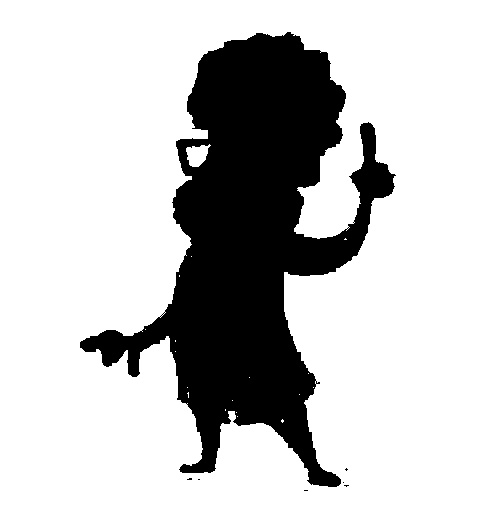注意：这里获取的mask为背景的mask，我们要获得人物的mask。
(3)获取人物mask
通过逻辑非操作取反，即可获得人物的mask区域(白色区域)：
mask = cv.bitwise_not(mask)
cv.imshow('mask2', mask)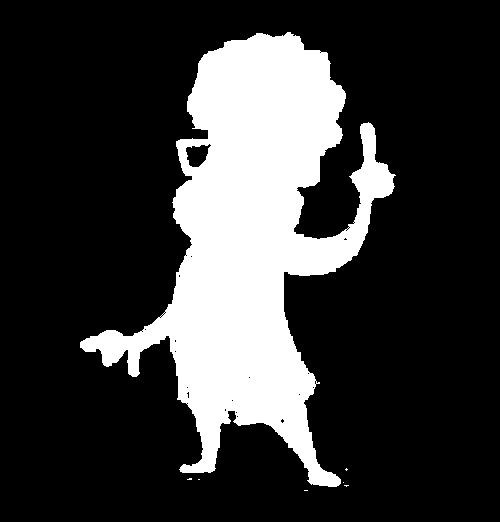(4)获取人物
将原始图像与原始图像在mask区域进行逻辑与操作，即可获取
timg1 = cv.bitwise_and(src, src, mask=mask)
cv.imshow('timg1', timg1)以上操作即提取了图像中的ROI(卡通人)区域，下面介绍将介绍将提取出来的图贴到其他背景上。
(5)新建一张与原始图一样大小的蓝色的背景图
background = np.zeros(src.shape, src.dtype)
background[:,:,0] = 255

(6)得到蓝色背景的mask
mask = cv.bitwise_not(mask)
dst = cv.bitwise_or(timg1, background, mask=mask)
cv.imshow('dst1', dst)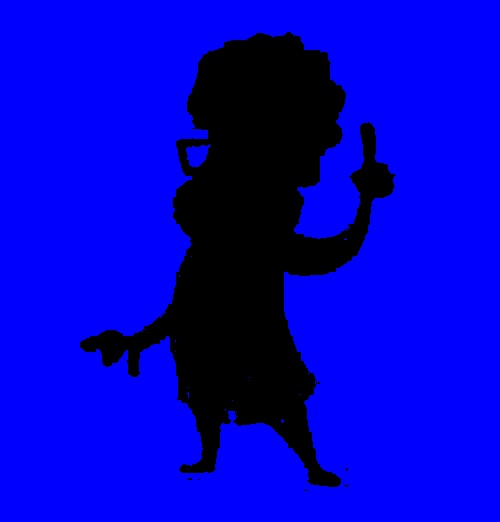(7)将人物图贴到蓝色背景上
dst = cv.add(dst, timg1)
cv.imshow('dst2', dst)4、整体代码
import cv2 as cv
import numpy as np

src = cv.imread('person.jpg')
cv.imshow('src', src)
hsv = cv.cvtColor(src, cv.COLOR_BGR2HSV)       # 转换成hsv色彩风格
mask = cv.inRange(hsv, (35, 43, 46), (99, 255, 255))        # 利用inRange产生mask
cv.imshow('mask1', mask)
cv.imwrite('mask1.jpg', mask)

# 获取mask
mask = cv.bitwise_not(mask)
cv.imshow('mask2', mask)
cv.imwrite('mask2.jpg', mask)
timg1 = cv.bitwise_and(src, src, mask=mask)
cv.imshow('timg1', timg1)
cv.imwrite('timg1.jpg', timg1)

# 生成背景
background = np.zeros(src.shape, src.dtype)
background[:,:,0] = 255

# 将人物贴到背景中
mask = cv.bitwise_not(mask)
dst = cv.bitwise_or(timg1, background, mask=mask)
cv.imshow('dst1', dst)
cv.imwrite('dst1.jpg', dst)

dst = cv.add(dst, timg1)
cv.imshow('dst2', dst)
cv.imwrite('dst2.jpg', dst)

cv.waitKey(0)
cv.destroyAllWindows()

参考
OpenCV学习笔记——HSV颜色空间超极详解&inRange函数用法及实战


展开全文数字图像处理 OpenCV python
• 首先利用dlib库识别出了人脸区域，现在要将识别出来的人脸区域进行保存，即提取ROI区域： 代码原来是利用便利的方式，写了两层循环来给新图像赋值 for ii in range(height * 2): for jj in range(width * 2): ...
• 图像感兴趣区域ROI提取主要使用掩模来进行。掩模是二值图像，感兴趣区域的掩模值设置为255，非感兴趣区域的掩模值为0 获取掩模的方法主要有两种 方法一 使用opencv中Mat函数方法,调用Mat(Rect).setTo方法设置...
• halcon软件是机器视觉图像处理函数库，资源便是通过halcon软件去提取ROI的源程序区域
• 本篇博文用于记录 cv::Mat 掩膜操作的学习心得，并附上一种基于掩膜操作的多边形roi区域提取方法。 cv::Mat 的掩膜操作(mask) 这里介绍cv::Mat常用的两个掩膜操作方法： void cv::Mat::setTo(inputArray array, ...opencv
• ## Halcon 提取ROI区域

万次阅读 2017-09-09 21:33:18
因reduce_domain提取的图像的大小与原图像大小保持一致，虽然可以结合crop_domain一起使用，去掉没有用到的区域； 但因reduce_domain提取的图像与实际所要的区域有一定区别(注：本人测试过程中，虽然提取的是原图像...
• 提取ROI区域 方法一： 方法二： 实际应用演示 原文出处： https://mp.weixin.qq.com/s/MiHz2zLBif_s1lksQXLBbw 微信公众号：OpenCV学堂 关注获取更多计算机视觉与深度学习知识 什么是ROI ROI是英文Region Of...opencv
• ## ENVI提取ROI区域步骤

千次阅读 热门讨论 2020-03-22 17:41:28
ENVI（The Environment for Visualizing Images）是一个完整的遥感图像处理平台，集图像数据的输入/输出、图像定标、图像增强、纠正、正射校正、镶嵌、数据融合以及各种变换、信息提取、图像分类等于一体。...机器学习 神经网络
• 对细胞与医疗图像来说，ROI提取正确才可以进行后续的分析、测量、计算密度等，而且这些ROI区域往往不是矩形区域，一般都是不规则的多边形区域，很多OpenCV初学者都不知道如何提取这些不规则的ROI区域。其实OpenCV中...
• #include #include #include #include #include using namespace std; using namespace cv; //全局变量定义 bool a(false); ...//定义感兴趣区域 void SetROI(Mat srcimage) { if(!a) { cout...